# Sport Climbing at Tokyo 2020, Part II: Competition Format

Who’s Suffering? Who’s Relevant?

Quang Nguyen https://github.com/qntkhvn
08-01-2021

This is the second post in a series about sport climbing at the 2020 Summer Olympics. In the first post, I performed a simulation study to examine scoring and rankings in sport climbing. This time, using data from major sport climbing competitions in recent years, I’m going to dive into the combined competition format and find out whether this format is appropriate for climbing athletes.

## Background

After the announcement of the decision to combine the three climbing disciplines (speed climbing, bouldering, lead climbing) at the 2020 Summer Olympics and only award one set of medals for each gender competition, the climbing world strongly reacted. Numerous climbers all over the world spoke out and shared their concerns about this new competition format. Here are some quotes from a series of Climbing Magazine interviews in 2016:

“I am NOT in support of the format that imposes that all climbers must compete in speed climbing. That is like asking a middle distance runner to compete in the sprint. Speed climbing is a sport within our sport.” - Lynn Hill

“Though sport and bouldering are more similar than speed, they still are different disciplines. Speed climbers will have the biggest disadvantage because their realm isn’t based on difficult movements.” - Courtney Woods

“Honestly, the people that will suffer the most are the ones that focus only on speed climbing. Those skills/abilities don’t transfer as well to the other disciplines.” - Mike Doyle

Other climbers used words and phrases like “bogus”, “a bummer”, “less than ideal”, and “cheesy and unfair” to describe the new format. A number of them also called for changes in future competitions, with suggestions like giving each event its own set of medals, or excluding speed and only including bouldering and lead.

Undoubtedly, the combined format decision received lots of thumbs-down. The question here is: Are the concerns about the new format valid? Let’s use statistics to find out.

## Who’s Suffering?

First, I’m going to look at the correlations between the overall rank and the ranks of the disciplines (speed, bouldering, and lead). I scraped data from the 2018 Youth Olympics (the scraping code can be found here if you’re interested), and computed Kendall’s tau (a nonparametric measure of ordinal association) for the ranked variables in the data. In the analysis that follows, I’m going to use data from the qualification rounds of both men’s and women’s competitions at the 2018 Youth Olympics.

Show code
``````library(tidyverse)
library(kableExtra)
theme_set(theme_light())

library(GGally)
mq %>%
select(Overall = rank, Speed = speed, Bouldering = bould, Lead = lead) %>%
ggpairs(diag = "blank",
axisLabels = "none",
upper = list(continuous = wrap("cor", method = "kendall", stars = FALSE))) +
theme(panel.grid.major = element_blank())
``````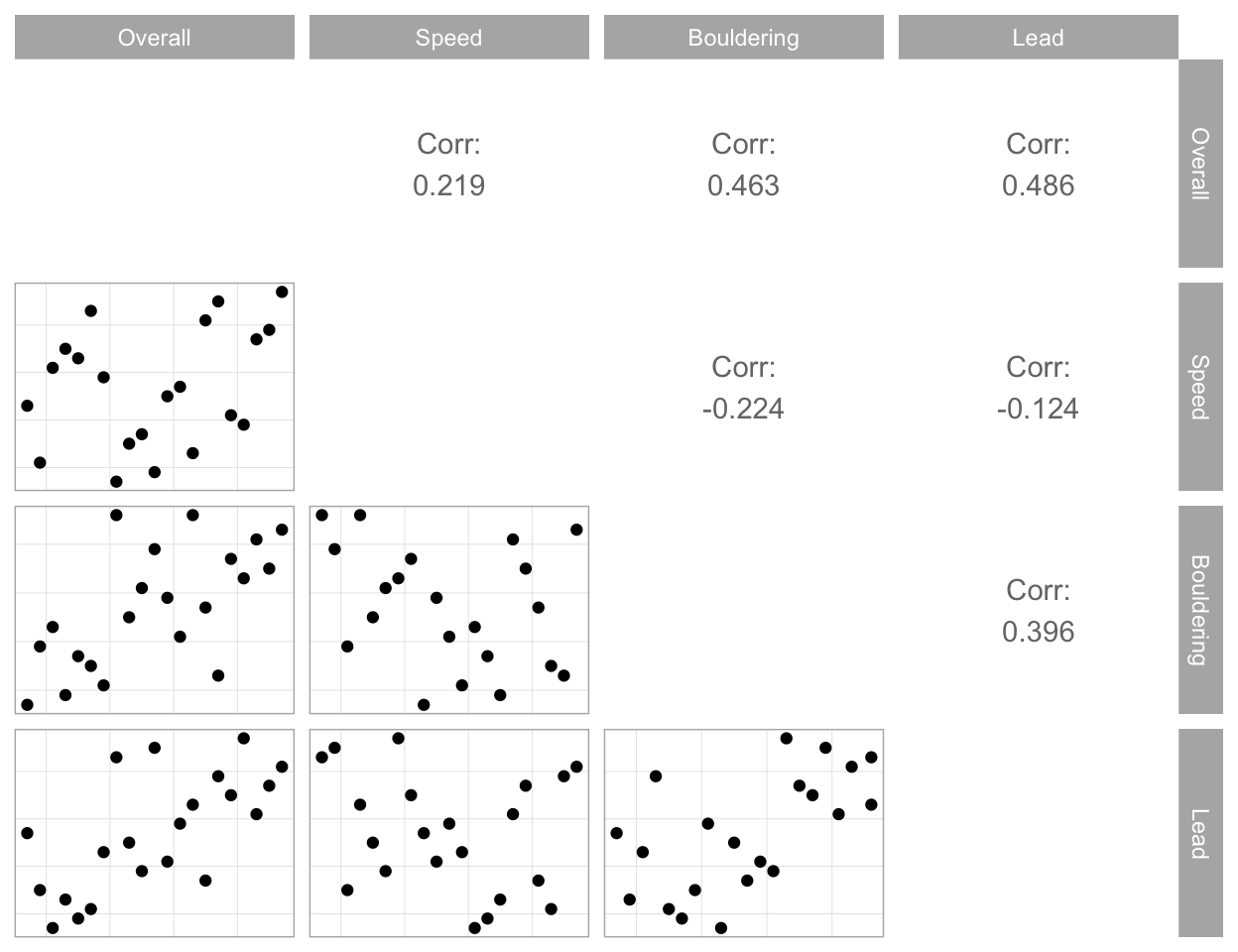Show code
``````wq %>%
select(Overall = rank, Speed = speed, Bouldering = bould, Lead = lead) %>%
ggpairs(diag = "blank",
axisLabels = "none",
upper = list(continuous = wrap("cor", method = "kendall", stars = FALSE))) +
theme(panel.grid.major = element_blank())
``````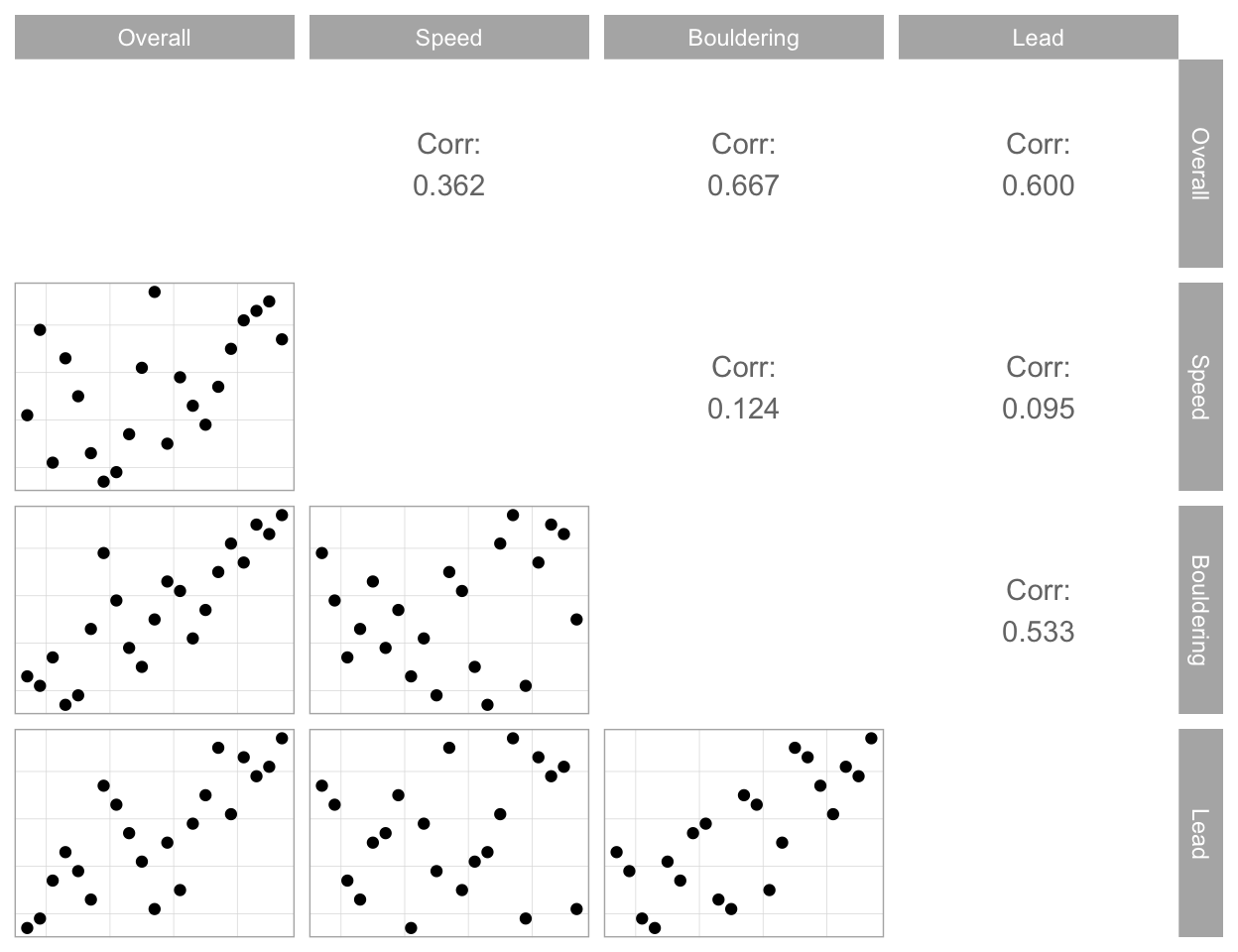From the figures above, there’s a fairly strong and positive relationship between bouldering and lead climbing ranks. These two variables are also highly correlated with the final ranking. On the flip side, the speed rank doesn’t seem to have much of a correlation with the other two events’ as well as with the overall rank. The climbers were absolutely right. As they pointed out in the interviews mentioned earlier, the three different climbing disciplines demand different sets of skills and, often, athletes specialize in a single event. Including speed climbing with bouldering and lead climbing is not the best idea. The idea of a climbing triathlon format gives a major disadvantage to speed climbers.

Speaking of speed climbing, after the first blog post was shared on Twitter, @jchaskell2 replied with a suggestion:

Have you looked at what the expected placement is for someone who goes 1x8x8 in the final? This is a legit likely score for the top speed climber.

This was brought up because in my previous post, I did some analyses on the probability of winning a medal given that a climber wins the first event, which happens to be speed climbing at this year’s Olympics. I went and ran a quick simulation study, where for each simulation, I first simulated one climber with the 1-8-8 scoring scheme, and then uniformly assigned the ranks for the remaining athletes.

Show code
``````set.seed(1)
nsim <- 10000
nplay <- 8

spec <- tibble(e1 = rep(1, nsim),
e2 = rep(nplay, nsim),
e3 = rep(nplay, nsim),
sim = 1:nsim) %>%
group_by(sim) %>%
mutate(player = sample(1:nplay, 1, replace = TRUE)) %>%
ungroup()

sim <- tibble(player = 1:nplay) %>%
slice(rep(row_number(), nsim)) %>%
mutate(sim = rep(1:nsim, each = nplay)) %>%
anti_join(spec) %>%
group_by(sim) %>%
mutate(e1 = sample(2:nplay, replace = FALSE),
e2 = sample(1:(nplay - 1), replace = FALSE),
e3 = sample(1:(nplay - 1), replace = FALSE)) %>%
ungroup() %>%
bind_rows(spec) %>%
arrange(sim, player) %>%
mutate(score = e1 * e2 * e3) %>%
group_by(sim) %>%
mutate(rank = rank(score, ties.method = "random")) %>%
ungroup()

res <- sim %>%
filter(e1 == 1 & e2 == 8 & e3 == 8) %>%
count(rank) %>%
mutate(prob = n / sum(n),
cum_prob = cumsum(prob))
``````

Here are numerical and visual summaries of the simulated data.

Show code
``````res %>%
kable()
``````
rank n prob cum_prob
2 3 0.0003 0.0003
3 292 0.0292 0.0295
4 2778 0.2778 0.3073
5 4874 0.4874 0.7947
6 1921 0.1921 0.9868
7 132 0.0132 1.0000
Show code
``````res %>%
mutate(rank = factor(rank)) %>%
ggplot(aes(x = rank, y = n)) +
geom_col() +
coord_flip() +
geom_text(aes(label = prob), hjust = -0.1, size = 3) +
expand_limits(y = 5010) +
ggtitle("Rank distribution for 1-8-8 climbers")
``````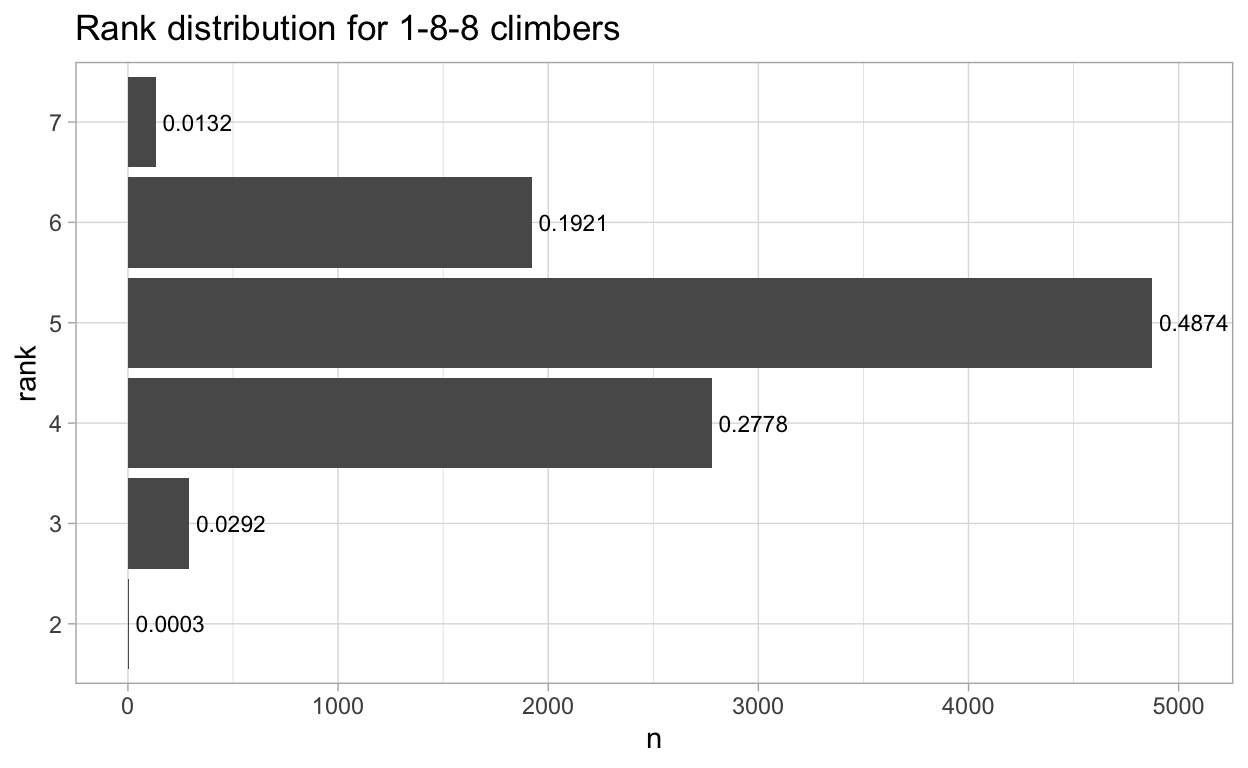Sure enough, being good at speed climbing doesn’t guarantee you a top 3 finish. In fact, according to my simulations, the chance of winning a medal for a 1-8-8 combination is very small - only 2.95%. Moreover, in almost half of the simulations, a 1-8-8 climber got 5th position in the standings.

Show code
``````res %>%
summarize(expected_rank = sum(rank * prob)) %>%
kable()
``````
expected_rank
4.8814

Finally, to answer @jchaskell2’s initial question, the expected placement for an athlete that goes 1-8-8 in the finals is 4.88, just slightly below 5, which doesn’t put them in a medalist position. I did mention the importance of getting off to a great start under this combined competition format in my simulation blog post. Well, this extreme case is an example where a perfect start isn’t good enough.

## Who’s Relevant?

This section is motivated by one question: What would happen to the rankings if one climber was left out?

The idea of Independence of Irrelevant Alternatives (IIA) comes up here. In layman’s terms, IIA is a rule which states that the results (rankings) of a competition should not change if a losing party is excluded.

Let’s continue to use data from the 2018 Youth Olympics, but this time I’m going to examine the final rankings of both male and female events. The data manipulating process here is very simple: after an athlete is dropped, the new ranks for each discipline of the remaining players are calculated. The new final scores can then be obtained (by multiplying the three event ranks), which determines the new overall ranks.

Show code
``````wf <- read_csv("https://raw.githubusercontent.com/qntkhvn/climbing/main/data/2018_youth_olympics/women_final.csv")

drop_rerank <- function(df) {
rerank <- list()
for (i in 1:nrow(df)) {
rerank[[i]] <- df[-i, ] %>%
mutate(rank_drop = i)
}

rerank_df <- df %>%
mutate(rank_drop = 0) %>%
bind_rows(rerank) %>%
group_by(rank_drop) %>%
mutate(
speed = rank(speed),
bould = rank(bould),
total = speed * bould * lead
) %>%
arrange(total, .by_group = TRUE) %>%
ungroup() %>%
group_by(rank_drop, total) %>%
mutate(
speed_tb = ifelse(speed < lag(speed), 1, 0),
bould_tb = ifelse(bould < lag(bould), 1, 0),
tb = speed_tb + bould_tb + lead_tb,
tb = ifelse(is.na(tb), 1, tb)
) %>%
ungroup() %>%
group_by(rank_drop) %>%
arrange(total,-tb, .by_group = TRUE) %>%
mutate(rank = row_number(),
last = str_to_title(last))

return(rerank_df)
}
``````

The figures below shows the changes to orderings of the men’s and women’s rankings when one climber is excluded. The panel number represents the rank of the contestant that was left out, with 0 being the actual standings of finalists. Instances where a change in rankings occurred are each highlighted by a black rectangular plot border, whereas players experiencing a position change are highlighted by red-filled bars.

Show code
``````drop_rerank(mf) %>%
mutate(last = fct_reorder(last, -rank),
rank = as.factor(rank),
rank_change = ifelse(
rank_drop %in% c(0, 4) |
rank_drop == 1 & rank %in% 4:5 |
rank_drop == 2 & rank %in% c(1, 5) |
rank_drop %in% c(3, 5) & rank %in% c(1, 2, 5) |
rank_drop == 6 & rank %in% 1:3,
"no", "yes")) %>%
ggplot(aes(x = last, y = total, fill = rank_change)) +
geom_col(show.legend = FALSE) +
geom_text(aes(label = rank), hjust = -0.2, size = 3) +
coord_flip() +
geom_rect(aes(xmin = -Inf, xmax = Inf, ymin = -Inf, ymax = Inf),
data = ~ filter(., rank_drop %in% c(1, 2, 3, 5, 6)),
color = "black", size = 1.5, fill = NA, inherit.aes = FALSE) +
facet_wrap(~ rank_drop, nrow = 2) +
expand_limits(y = 102, x = 0:7) +
scale_fill_manual(values = c("grey", "chocolate")) +
labs(y = "Score",
x = "Climber",
title = "Leave-one-climber-out Plot - 2018 Youth Olympics Men's Competition") +
theme(axis.ticks = element_blank(),
panel.grid.minor = element_blank())
``````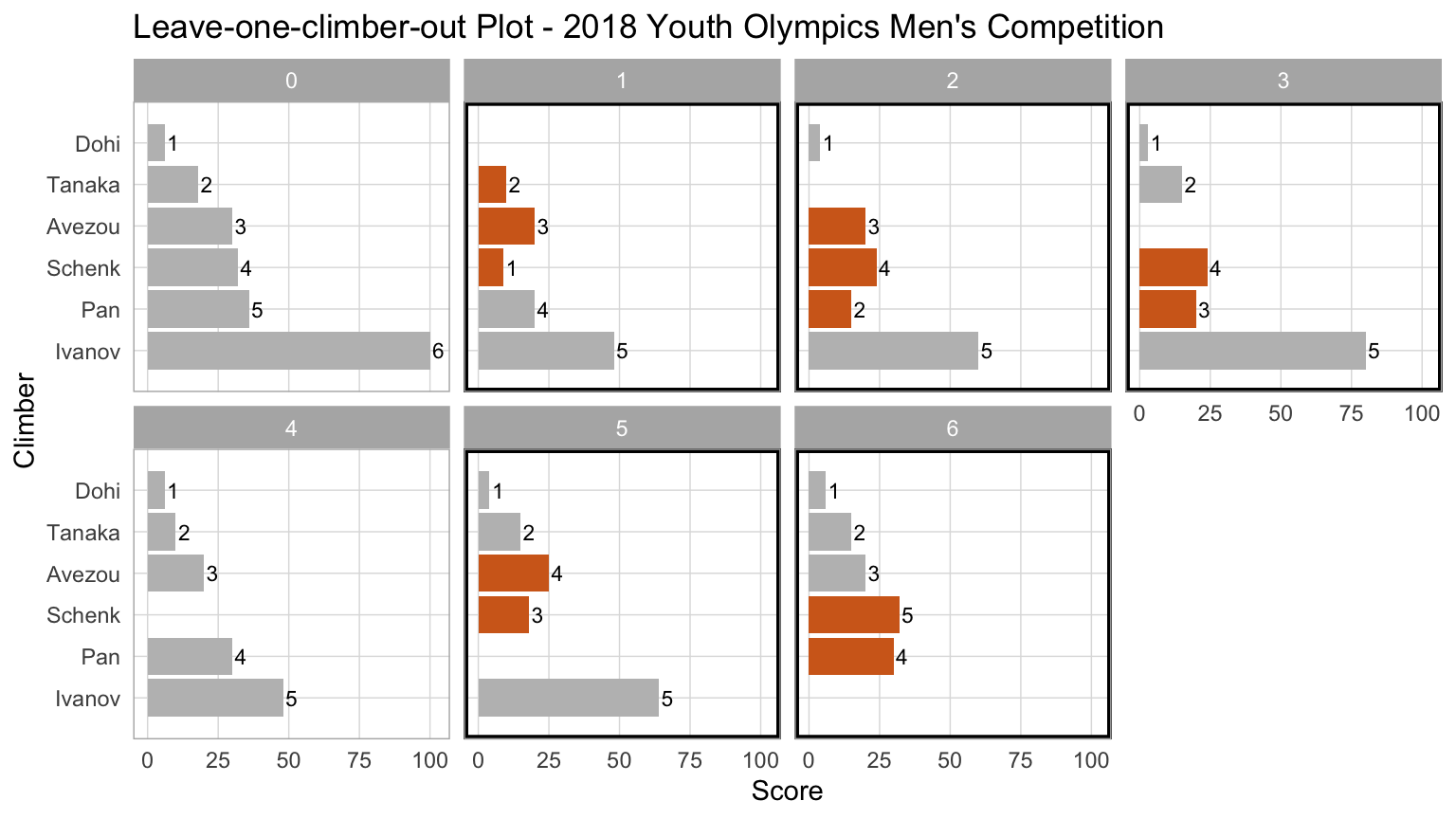Show code
``````drop_rerank(wf) %>%
mutate(last = fct_reorder(last, -rank),
rank = as.factor(rank),
rank_change = ifelse(
rank_drop %in% c(0, 1, 4, 6) |
rank_drop == 2 & rank %in% 1:3 |
rank_drop == 3 & rank %in% c(1, 4, 5) |
rank_drop == 5 & rank %in% c(1, 5),
"no", "yes")) %>%
ggplot(aes(x = last, y = total, fill = rank_change)) +
geom_col(show.legend = FALSE) +
geom_text(aes(label = rank), hjust = -0.2, size = 3) +
coord_flip() +
geom_rect(aes(xmin = -Inf, xmax = Inf, ymin = -Inf, ymax = Inf),
data = ~ filter(., rank_drop %in% c(2, 3, 5)),
color = "black", size = 1.5, fill = NA, inherit.aes = FALSE) +
facet_wrap(~ rank_drop, nrow = 2, ncol = 4) +
expand_limits(y = 62, x = 0:7) +
scale_fill_manual(values = c("grey", "red")) +
labs(y = "Score",
x = "Climber",
title = "Leave-one-climber-out Plot - 2018 Youth Olympics Women's Competition") +
theme(axis.ticks = element_blank(),
panel.grid.minor = element_blank())
``````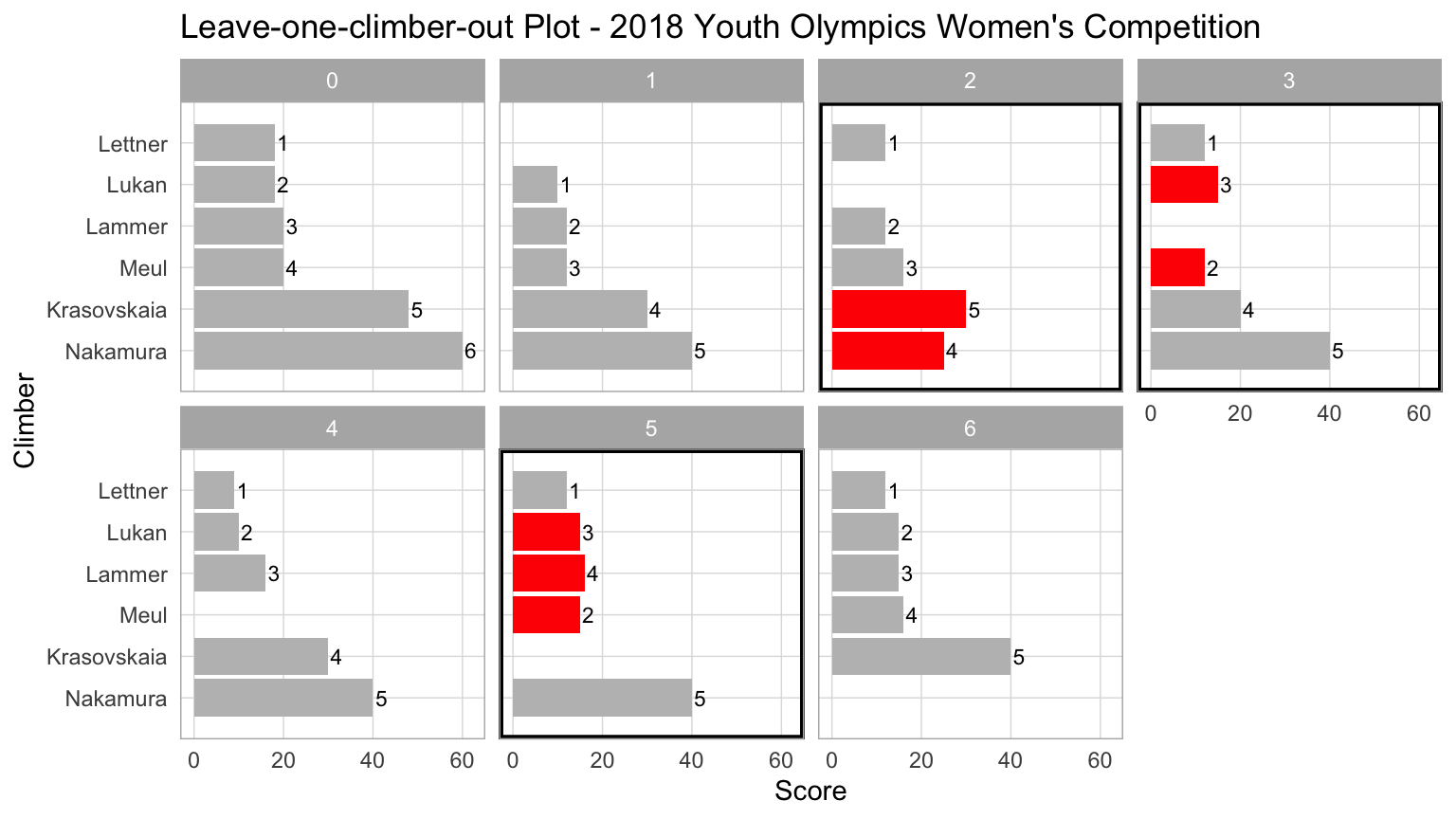There are two very interesting cases here, where significant changes happened to the original rankings:

A lower-ranked climber is excluded (IIA)

Panel 5 of the women’s event is a perfect example of this situation. If fifth-ranked Krasovskaia was dropped, Meul, who finished fourth overall in real life, would jump two spots to take the silver medal. Bronze medalist Lammer would now be in fourth place. The IIA criterion is violated.

A higher-ranked climber is excluded

Panels 1 and 2 of the men’s competition are good illustrations of this case. If first-ranked Dohi was excluded, Schenk, whose actual rank was fourth and did not earn a medal, would suddenly move up to claim gold. How wild! Similarly, if the silver medal recipient Tanaka was left out, fifth-ranked Pan would leap ahead two spots to second and win silver.

## Takeaways

This format (competition + scoring) is certainly not the best idea. Combining speed climbing, bouldering, and lead climbing isn’t a good idea either. Speed climbing needs to be separated and have its own set of medals.

By the way, there’s a paper in progress. As always, comments and suggestions are greatly appreciated.

### Citation

`Nguyen (2021, Aug. 1). The Q: Sport Climbing at Tokyo 2020, Part II: Competition Format. Retrieved from https://qntkhvn.netlify.app/posts/2021-07-31-climbing-p1-sim`
```@misc{nguyen2021sport,